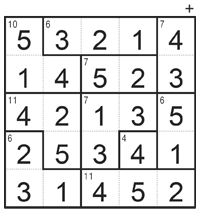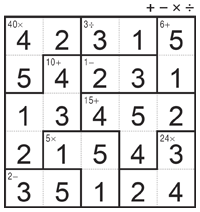﻿ CalcuDoku правила
 ВходРегистрация# CalcuDoku правила

Following the footsteps of Sudoku, Kakuro and other Number Logic puzzles, CalcuDoku is one more family of easy to learn addictive logic puzzles which were invented in Japan. Using logic together with the four math operations, these fascinating puzzles offer endless fun and intellectual entertainment to puzzle fans of all skills and ages.

CalcuDoku are math based puzzles coupled with logic. Unlike other logic puzzles, CalcuDoku uses addition, subtraction, multiplication and division in ways which are deeper and more gratifying than anyone can imagine.

CalcuDoku puzzles come in many sizes and range from very easy to extremely difficult taking anything from five minutes to several hours to solve. However, make one mistake and you’ll find yourself stuck later on as you get closer to the solution...

If you like Sum Sudoku, Kakuro and other logic puzzles, you will love Conceptis CalcuDoku as well!

## SingleOp CalcuDoku

Each puzzle consists of a grid containing blocks surrounded by bold lines. The object is to fill all empty squares so that the numbers 1 to N (where N is the number of rows or columns in the grid) appear exactly once in each row and column and the numbers in each block produce the result shown in the top-left corner of the block according to the math operation appearing on the top of the grid. In CalcuDoku a number may be used more than once in the same block.## DualOp CalcuDoku

Each puzzle consists of a grid containing blocks surrounded by bold lines. The object is to fill all empty squares so that the numbers 1 to N (where N is the number of rows or columns in the grid) appear exactly once in each row and column and the numbers in each block produce the result of the math operation shown in the top-left corner of the block. In CalcuDoku a number may be used more than once in the same block.